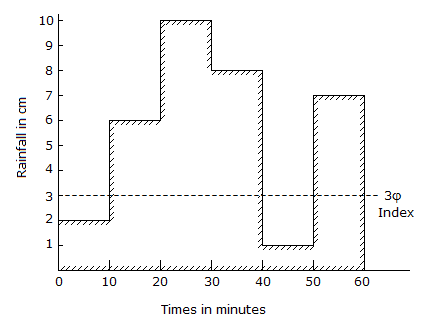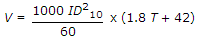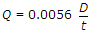Courses

# Test: Water Resources Engineering - 4

## 25 Questions MCQ Test Water Resources Engineering | Test: Water Resources Engineering - 4

Description
This mock test of Test: Water Resources Engineering - 4 for Civil Engineering (CE) helps you for every Civil Engineering (CE) entrance exam. This contains 25 Multiple Choice Questions for Civil Engineering (CE) Test: Water Resources Engineering - 4 (mcq) to study with solutions a complete question bank. The solved questions answers in this Test: Water Resources Engineering - 4 quiz give you a good mix of easy questions and tough questions. Civil Engineering (CE) students definitely take this Test: Water Resources Engineering - 4 exercise for a better result in the exam. You can find other Test: Water Resources Engineering - 4 extra questions, long questions & short questions for Civil Engineering (CE) on EduRev as well by searching above.
QUESTION: 1

Solution:
QUESTION: 2

Solution:
QUESTION: 3

### The rate of rainfall for successive 10 minute periods of a 60 minute duration storm, are shown in the below figure. If the value of φindex is 3 cm/hour, the run off will beSolution:
QUESTION: 4

Izzard formula for the time of concentration in minutes for the plots having no channels, is (where Lo is the length of overland flow in metres and Kp rainfall intensity in cm/hour)

Solution:
QUESTION: 5

From the data of the rain storm shown in the below figure, the value of Windex isSolution:
QUESTION: 6

A unit hydrograph is a hydrograph of a rain storm of a specified duration resulting from a run-off of

Solution:
QUESTION: 7

Pick up the correct statement from the following :

Solution:
QUESTION: 8

The best unit period of a unit hydrograph, is equal to basin lag divided by

Solution:
QUESTION: 9

If total run off of a basin of 50 hectares is 0.8 cm, the ordinate of Bernard's distribution graph, may be calculated by the formula (where Q is the discharge in cumecs at the given time)

Solution:

Run off Q= CIA.
= 6.25*50*0.8.
C=I/A=50/8 = 250 8 because in metre,
= 250.

QUESTION: 10

Pick up the correct statement from the following :

Solution:
QUESTION: 11

If the grain size of soil increases

Solution:
QUESTION: 12

The equationwhich is used for determining the velocity of ground water flow in metres per day is known as

Solution:
QUESTION: 13

Pick up the correct statement from the following :

Solution:
QUESTION: 14

While determining the yield of a gravity well by pumping, the depth of water table in two tests wells at distances r1 and r2 from the centre of the main well were found to be s1 and s2 respectively. Assuming the coefficient of transmissibility of the soil as T, the discharge Q may be given by

Solution:
QUESTION: 15

According to Thiem, the permeability of an aquifer may be obtained from the equation

Solution:
QUESTION: 16

The Dupuit formula is based on

Solution:
QUESTION: 17

A well is sunk in an unconfined aquifer having a saturated depth of 100 m. Assuming the equilibrium flow conditions and a homogeneous aquifer and radius of influence to be same, the ratio of discharges at 20 m and 40 m draw downs, is

Solution:
QUESTION: 18

Evaporation losses depend upon

Solution:
QUESTION: 19

Phytometer method is generally used for the measurement of

Solution:
QUESTION: 20

In the estimate of design flood, Dickens assumes that high flood in cumecs, is proportional to catchment area raised to the power

Solution:
QUESTION: 21

For high flood estimates the average value of the constant C in Dicken's formula Q = CA3/4, is

Solution:
QUESTION: 22

Pettis formula Q = C (P.B)5/4 cumecs, is based upon

Solution:
QUESTION: 23

Boston society of Civil Engineer's formulain cumecs/square km is based upon

Solution:
QUESTION: 24

For predicting floods of a given frequency, the best reliable method is

Solution:
QUESTION: 25

The earthen embankments constructed parallel to the river banks at some suitable distance for flood control, are known as

Solution: